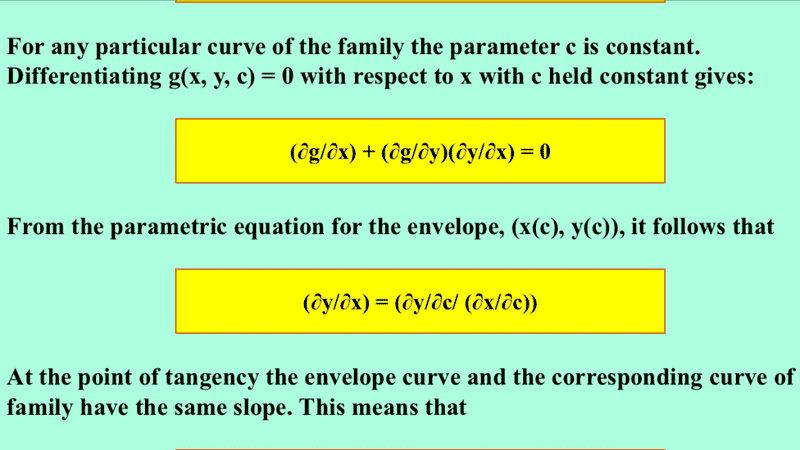# Derive the formula for gradient using chain rule

• I
Consider a surface defined by the equation ##g(x, y, z)=0##. The intersection between this surface and the plane ##z=c## produces a curve that can be plotted on an x-y plane. Find the gradient of this curve.

By chain rule,

##\frac{\partial y}{\partial x}=\frac{\partial y}{\partial g}\frac{\partial g}{\partial x}##

Using the reciprocity relation ##\frac{\partial y}{\partial g}=\Big(\frac{\partial g}{\partial y}\Big)^{-1}##, we have

##\frac{\partial y}{\partial x}=\frac{(\frac{\partial g}{\partial x})}{(\frac{\partial g}{\partial y})}##

This differs from the correct answer by a negative sign. What's wrong with this method?

andrewkirk
Homework Helper
Gold Member
Greater care is needed when handling partial derivatives. They cannot be treated in general the same as total derivatives. In particular it is not necessarily the case that
##\frac{\partial y}{\partial x}=\frac{\partial y}{\partial g}\frac{\partial g}{\partial x}##
Indeed, in this case the ##\frac{\partial y}{\partial x}## that is being calculated is with the values of ##g## and ##z## held constant, so ##\frac{\partial y}{\partial g}## is undefined or infinite, while ##\frac{\partial g}{\partial x}## is zero.

•Happiness
The following website calculates the gradient as follows:

Differentiate ##g## wrt ##x## while holding ##z## constant.Then we will have ##\frac{\partial y}{\partial x}=-\frac{(\frac{\partial g}{\partial x})}{(\frac{\partial g}{\partial y})}##. Why ain't ##\frac{\partial g}{\partial x}## and ##\frac{\partial g}{\partial y}## zero in this case?

In what situations must the two partials on the RHS of the chain rule have the same variables held constant? Like you mentioned for post #1, ##(\frac{\partial y}{\partial g})_{gz}(\frac{\partial g}{\partial x})_{gz}##. But why is it not required in the attachment above, where ##(\frac{\partial g}{\partial y})_{xz}(\frac{\partial y}{\partial x})_{gz}##?

Source:
http://www.sjsu.edu/faculty/watkins/envelopetheo.htm

Last edited:
andrewkirk
Homework Helper
Gold Member
I find the presentation on that web page unnecessarily confusing. It attempts to use the formula for a total derivative in a case where all derivatives are partial. Sometimes one can get away with this, but sometimes one can't, so it is best avoided.

A more correct derivation would be as follows:

Say the point ##(x_0,y_0)## is on the curve. Let us parametrise the curve through that point by function ##\gamma:\mathbb R\to\mathbb R^2## and wlog set ##\gamma(0)=(x_0,y_0)##. Then we have ##g(\gamma_1(t).\gamma_2(t),c)=0##, where ##\gamma_1## and ##\gamma_2## are the component functions of ##\gamma##.

We then apply ##\frac d{dt}## to this - ie taking a total derivative - to get:

$$0=\frac d{dt}0=\frac d{dt}g(\gamma_1(t).\gamma_2(t),c)=\frac{\partial g}{\partial x}\frac {dx}{dt}+ \frac{\partial g}{\partial y}\frac {dy}{dt}+\frac{\partial g}{\partial c}\frac {dc}{dt} =\frac{\partial g}{\partial x}\frac {dx}{dt}+ \frac{\partial g}{\partial y}\frac {dy}{dt}+\frac{\partial g}{\partial c}\cdot 0$$

Rearranging, we get

$$-\frac{\partial g}{\partial x}/\frac{\partial g}{\partial y}=\frac{dy}{dt}/\frac{dx}{dt}=\frac{dy}{dx}$$
as required. Note that the transformation in the final step is allowed because we are dealing with total derivatives, not partial derivatives.

•Happiness and Delta2